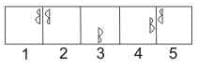JEE  >  Test: BITSAT Mock Test- 2

# Test: BITSAT Mock Test- 2

Test Description

## 150 Questions MCQ Test | Test: BITSAT Mock Test- 2

Test: BITSAT Mock Test- 2 for JEE 2023 is part of JEE preparation. The Test: BITSAT Mock Test- 2 questions and answers have been prepared according to the JEE exam syllabus.The Test: BITSAT Mock Test- 2 MCQs are made for JEE 2023 Exam. Find important definitions, questions, notes, meanings, examples, exercises, MCQs and online tests for Test: BITSAT Mock Test- 2 below.
Solutions of Test: BITSAT Mock Test- 2 questions in English are available as part of our course for JEE & Test: BITSAT Mock Test- 2 solutions in Hindi for JEE course. Download more important topics, notes, lectures and mock test series for JEE Exam by signing up for free. Attempt Test: BITSAT Mock Test- 2 | 150 questions in 180 minutes | Mock test for JEE preparation | Free important questions MCQ to study for JEE Exam | Download free PDF with solutions
 1 Crore+ students have signed up on EduRev. Have you?
Test: BITSAT Mock Test- 2 - Question 1

### If an 8Ω resistance and 6Ω reactance are present in an AC series circuit then the impedence of the circuit will be

Detailed Solution for Test: BITSAT Mock Test- 2 - Question 1

Solution :- Z^2 = R^2 + X^2

Z = [(8)^2 + (6)^2]^½

Z = ^½

Z = 10 ohm

Test: BITSAT Mock Test- 2 - Question 2

### If the energy of hydrogen atom in ground state is -13.6 eV, then its energy in the first excited state will be

Test: BITSAT Mock Test- 2 - Question 3

### In which radioactive disintegration, neutron dissociates into proton and electron

Test: BITSAT Mock Test- 2 - Question 4

The appropriate material to be used in the construction of resistance boxes out the following is

Test: BITSAT Mock Test- 2 - Question 5

The resistance of an ammeter is 13 Ω and its scale is graduated for a current upto 100A. After an additional shunt has been connected to this ammeter it becomes possible to measure currents upto 750A by this meter. The value of shunt resistance is

Test: BITSAT Mock Test- 2 - Question 6

An oxide coated filament is useful in vacuum tubes because essentially

Test: BITSAT Mock Test- 2 - Question 7

In Thomson experiment of finding e/m for electrons, beam of electron is replaced by that of muons (particle with same charge as of electrons but mass 208 times that of electrons). No deflection condition in this case satisfied if

Test: BITSAT Mock Test- 2 - Question 8

What is the unit of self inductance of a coil ?

Test: BITSAT Mock Test- 2 - Question 9

A 80 μF capacitor in a defibrillator is charged to 100 V. The energy stored in the capacitor is sent through the patient during a pulse of duration 2 ms. The power delivered to the patient is

Test: BITSAT Mock Test- 2 - Question 10

The capacitance of a parallel plate capacitor is 10 μF when distance between its plates is 8 cm. If distance between the plates is reduced to 4 cm, its capacitance will be

Test: BITSAT Mock Test- 2 - Question 11

A satellite whose mass is M, is revolving in circular orbit of radius r aroud the earth. Time of revolution of satellite is

Detailed Solution for Test: BITSAT Mock Test- 2 - Question 11

Explanation : Let the satellite of mass ms is revolving around the Earth of mass me at a distance of r then the centripetal force will be given by:

Fc = msv2/r

where v is velocity of satellite. This force is equal to gravitational force between satellite and earth. Therefore

(msv2)/r = (Gmsme)/r2

v = (Gme/r)1/2

Now, period of the satellite is given by:

T = 2πr/v

= 2π(r3/Gme)1/2

​{2π is constant}

Therefore T is proportional to  2π(r3/Gme)1/2

Test: BITSAT Mock Test- 2 - Question 12

Pulling force making an angle θ to the horizontal is applied on a block of weight W placed on a horizontal table. If the angle of friction is α. then the magnitude of force required to move the body is equal to

Test: BITSAT Mock Test- 2 - Question 13

0.1m3 of water at 80o C is mixed with 0.3m3 of water at 60o C . The final temperature of the mixture is

Test: BITSAT Mock Test- 2 - Question 14

At a given volume and temperture, the pressure of a gas

Test: BITSAT Mock Test- 2 - Question 15

A cyclotron oscillator frequency is 10 MHz. If radius of its dees 60 cm, then magnetic field for accelerating the protons is (Given e = 1.6 x 10-19C, mp = 1.67 x 10-27 kg)

Detailed Solution for Test: BITSAT Mock Test- 2 - Question 15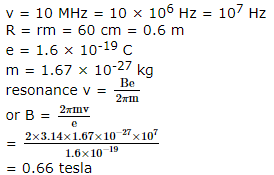Test: BITSAT Mock Test- 2 - Question 16

If a substance is to be paramagnetic, its atoms must have a

Test: BITSAT Mock Test- 2 - Question 17

The change in surface energy, when a drop of surface tension T and radius of R splits into 1000 droplets of radius r, is

Test: BITSAT Mock Test- 2 - Question 18

In a container, the velocity of water flowing through a hole at a depth h from the surface of water is 5 m-s⁻1. If the container has the hole at a depth 4h from the surface of water, then velocity of water flowing through the hole is

Test: BITSAT Mock Test- 2 - Question 19

A copper wire of length 4 m and area of cross-section 1.2 cm2 is stretched with a force of 4.8 x 103 N. If the Young's modulus for copper is 1.2 x 1011 N-m⁻2, then increase in the length of the wire will be

Test: BITSAT Mock Test- 2 - Question 20

A body, thrown upwards with some velocity, reaches the maximum height of 20 m. Another body with double the mass thrown up, with double initial velocity will reach a maximum height of

Test: BITSAT Mock Test- 2 - Question 21

Which of the following does not represent a zero vector?

Test: BITSAT Mock Test- 2 - Question 22

The parametric equations of a curve traced by a projectile on a certain planet with no surrounding are given by :y = (8t-5t2) metre and x = 6t metre, where t is in seconds. The velocity with which the projectile is projected, is

Test: BITSAT Mock Test- 2 - Question 23

The hypermetropia is a

Test: BITSAT Mock Test- 2 - Question 24

A parachutist of weight W strikes the ground with his logs fixed and comes to rest with an upward acceleration of magnitude '3g'. Force exerted on him by the ground during landing is

Test: BITSAT Mock Test- 2 - Question 25

The kinetic energy of a particle executing simple harmonic motion is 16 J when it is in its mean position. If the amplitude of oscillation is 25 cm and the mass of the particle is 5.12 kg, the tme period of its oscillation is

Test: BITSAT Mock Test- 2 - Question 26

The bob of a simple pendulum is a spherical hollow ball filled with water. A plugged hole near the bottom of the oscillating bob gets suddenly unplugged. During the observation, till water is coming out, the time period of oscillation would

Test: BITSAT Mock Test- 2 - Question 27

With respect to air, critical angle in a medium for light of red colour [λ₁] is θ. Other facts remaining same, critical angle for light of yellow colour [λ₂] will be

Test: BITSAT Mock Test- 2 - Question 28

A wheel having moment of inertia 2 kg-m2 about its vertical axis, rotates at the rate of 60 rev-min⁻1 about this axis. The torque, which can stop the wheel's rotation in one minute, would be

Test: BITSAT Mock Test- 2 - Question 29

A mass of 2 kg tied to a string of 1m length is rotated in a vertical circle with a uniform speed of 4ms-1. The tension in the string will be 52N, when the mass is at

Test: BITSAT Mock Test- 2 - Question 30

Before jumping in water from above, a swimmer bends his body to

Test: BITSAT Mock Test- 2 - Question 31

An electric lamp is marked 60 W, 230 V. The cost of 1 kilowatt hour of power is Rs. 1.25. The cost of using this lamp for 8 hours is

Test: BITSAT Mock Test- 2 - Question 32

A block of mass 100 gm slides on a rough horizontal surface. If the speed of the block decreases from 10 m/s to 5 m/s, the thermal energy developed in the process is

Test: BITSAT Mock Test- 2 - Question 33

In which process the P-V indicator diagram is a straight line parallel to volume axis

Test: BITSAT Mock Test- 2 - Question 34

If C and R denote the capacitance and resistance respectively, then the dimensional formula for CR is same as that fore

Detailed Solution for Test: BITSAT Mock Test- 2 - Question 34

CR is time constant of CR circuit

Test: BITSAT Mock Test- 2 - Question 35

Wave optics is based on wave theory of light put forward by Huygen and modified later by

Test: BITSAT Mock Test- 2 - Question 36

In the propagation of light waves, the angle between the direction of vibration and plane of polarisation is

Test: BITSAT Mock Test- 2 - Question 37

The velocity of waves in a string fixed at both ends is 2 m/s. The string forms standing waves with nodes 5.0 cm apart. The frequency of vibration of the string in Hz is

Test: BITSAT Mock Test- 2 - Question 38

Two bodies have their resonant frequencies at 252 and 256 υib/sec respectively. The beat frequency produced when they vibrate together is

Test: BITSAT Mock Test- 2 - Question 39

The earth's radius is R and acc. due to gravity at its surface is g. If a body of mass m falls from a height h = R/5 from earth's surface, its potential energy decreases by

Test: BITSAT Mock Test- 2 - Question 40

A motor pump set lifts 300 Kg of water per minute from a well of depth 20 m and delivers to a height of 20 m. Then its power is

Detailed Solution for Test: BITSAT Mock Test- 2 - Question 40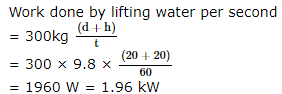Test: BITSAT Mock Test- 2 - Question 41

Major constituent of dynamite is

Test: BITSAT Mock Test- 2 - Question 42

The orientation of an atomic orbital is governed by

Test: BITSAT Mock Test- 2 - Question 43

The general order of reactivity of carbonyl compounds towards nucleophilic addition reactions is?

Test: BITSAT Mock Test- 2 - Question 44

In a given atom, no two electrons can have the same values of all the quantum numbers. This is called

Test: BITSAT Mock Test- 2 - Question 45

Homolytic fission of C - C bond in ethane gives an intermediate in which carbon is

Detailed Solution for Test: BITSAT Mock Test- 2 - Question 45

Homolytic fission of C — C bond gives free radicals in which carbon is sp2 hybridised.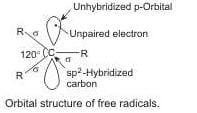Test: BITSAT Mock Test- 2 - Question 46

Which of the following has a derived unit?

Test: BITSAT Mock Test- 2 - Question 47

Which of the following is an electrophile?

Test: BITSAT Mock Test- 2 - Question 48

The effect of atoms or groups loosing electrons towads a carbon atom is called

Test: BITSAT Mock Test- 2 - Question 49

Reaction of O₂ and CO with haemoglobin gives

Test: BITSAT Mock Test- 2 - Question 50

Test: BITSAT Mock Test- 2 - Question 51

Carboxylic acids are more acidic than phenol and alcohol because of

Test: BITSAT Mock Test- 2 - Question 52

In diamond, the bonds present are

Test: BITSAT Mock Test- 2 - Question 53

A good fuel should have

Test: BITSAT Mock Test- 2 - Question 54

A reversible reaction is said to have attained equilibrium, when

Test: BITSAT Mock Test- 2 - Question 55

Al3+ has a lower ionic radius than Mg2+ ion because

Detailed Solution for Test: BITSAT Mock Test- 2 - Question 55

Al3+ has a higher nuclear charge (+ 13) and consequently the larger force of attraction by the nucleus for the valence shell electrons than that of Mg2+ (+ 12).

Test: BITSAT Mock Test- 2 - Question 56

A graph plotted between log k versus 1/T for calculating activation energy is shown by

Test: BITSAT Mock Test- 2 - Question 57

In an adiabatic expansion of ideal gas:

Test: BITSAT Mock Test- 2 - Question 58

Paracetamol is an

Test: BITSAT Mock Test- 2 - Question 59

The oxidation state of Fe in K₄[Fe(CN)₆] is

Test: BITSAT Mock Test- 2 - Question 60

The transition elements are more metallic than the representative elements, because they are

Test: BITSAT Mock Test- 2 - Question 61

Which of the following element belongs to the second row transition series?

Test: BITSAT Mock Test- 2 - Question 62

In which cell of the free energy of a chemical reaction is directly converted into electricity ?

Test: BITSAT Mock Test- 2 - Question 63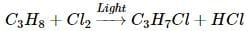The above reaction is an example of

Test: BITSAT Mock Test- 2 - Question 64

Benzene is obtained by fractional distillation of

Test: BITSAT Mock Test- 2 - Question 65

H₂O₂ is commonly prepared in lab. by the reaction of

Test: BITSAT Mock Test- 2 - Question 66

If the solubility of PbCl₂ at 25oC is 6.3 x 10⁻3 mole/litre, its solubility product at that temperature is

Test: BITSAT Mock Test- 2 - Question 67

Which of the following will have a meso-isomer?

Test: BITSAT Mock Test- 2 - Question 68

A compound A has a molecular formula C₂Cl₃OH. It reduces Fehling solution and on oxidation give a monocarboxylic acid B. A is obtained by the action of chlorine on ethyl alcohol. A is

Test: BITSAT Mock Test- 2 - Question 69

Nitrogen atom has an atomic number of 7 and oxygen has an atomic number 8. The total number of electrons in a nitrate ion will be

Test: BITSAT Mock Test- 2 - Question 70

Concentrated nitric acid oxidises phosphorus to

Test: BITSAT Mock Test- 2 - Question 71

Sea divers go deep into the sea water with a mixture of which of the following gases?

Test: BITSAT Mock Test- 2 - Question 72

Water gas is produced by

Test: BITSAT Mock Test- 2 - Question 73

FeSO₄ forms brown ring with

Test: BITSAT Mock Test- 2 - Question 74

F₂C = CF₂ is a monomer of

Test: BITSAT Mock Test- 2 - Question 75

Sodium is made by the electrolysis of a molten mixture of about 40% NaCl and 60% CaCl₂ because

Test: BITSAT Mock Test- 2 - Question 76

What are the products formed when Li2CO3 undergoes decomposition?

Test: BITSAT Mock Test- 2 - Question 77

When the solute is present in trace quantities, the following expression is used

Test: BITSAT Mock Test- 2 - Question 78

If two moles of an ideal gas at 546 K occupy volume of 44.8 L, then pressure must be

Detailed Solution for Test: BITSAT Mock Test- 2 - Question 78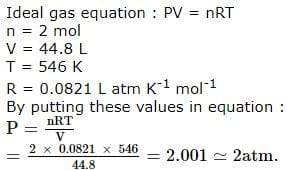Test: BITSAT Mock Test- 2 - Question 79

If 0.5g of metal on oxidation formed 0.79 g of its oxide, then equivalent weight of the metal is

Test: BITSAT Mock Test- 2 - Question 80

According to the adsorption theory of catalysis, the speed of the reaction increases because

Test: BITSAT Mock Test- 2 - Question 81

The area bounded by y = cos x, y = x + 1, y = 0 is

Test: BITSAT Mock Test- 2 - Question 82

If the sum of the coefficients in the expansion of (α2x2 - 2αx + 1)51 vanishes, then α is equal to

Test: BITSAT Mock Test- 2 - Question 83

The lines 3x - 4y + 4 = 0 and 6x - 8y - 7 = 0 are tangent to the same circle. The radius of this circle is

Test: BITSAT Mock Test- 2 - Question 84

If the circles x2 + y2 = 9 and x + y + 2ax + 2y + 1 = 0 touch each other, then a is

Test: BITSAT Mock Test- 2 - Question 85

The amplitude of sin(π/5) + i(1 - cos(π/5))is

Test: BITSAT Mock Test- 2 - Question 86

Which equation has the solution y=A sinx+B cosx?

Test: BITSAT Mock Test- 2 - Question 87

The solution of differential equation (dy/dx)=[(1+y2)/(1+x2)] is

Test: BITSAT Mock Test- 2 - Question 88

The solution of the equation ydx+(1+x2)tan⁻1xdy=0 is

Test: BITSAT Mock Test- 2 - Question 89

If y=(1+x1/4)(1+x1/2)(1-x1/4), (dy/dx)=

Test: BITSAT Mock Test- 2 - Question 90

(d/dx)[cos⁻1((x-x⁻1)/(x+x⁻1))]=

Test: BITSAT Mock Test- 2 - Question 91

Find the sum of the series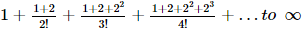Detailed Solution for Test: BITSAT Mock Test- 2 - Question 91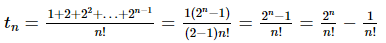Sum of the given series:Sum of the given series: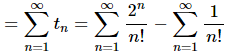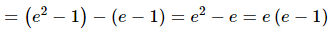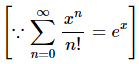Test: BITSAT Mock Test- 2 - Question 92

AB is a vertical pole. The end A is on the level ground, C is the middle point of AB and P is a point on the level ground. The portion CB subtends an angle β at P. If AP=nAB, tanβ=

Test: BITSAT Mock Test- 2 - Question 93

The line y = 4x + c touches the hyperbola x2 - y2 = 1 if

Test: BITSAT Mock Test- 2 - Question 94

The area between (x2/a2) + (y2/b2) = 1 and the straight line (x/a) + (y/b) = 1 is

Test: BITSAT Mock Test- 2 - Question 95

The area enclosed between the curve y2 = 4x and the line y = x is

Test: BITSAT Mock Test- 2 - Question 96

If f(x)=[(1-x)/(1+x)], then f[f(cos 2θ)]=

Test: BITSAT Mock Test- 2 - Question 97

If sin⁻1 x = π/5, for some x ∈[- 1, 1], then the value of cos⁻1 x is

Test: BITSAT Mock Test- 2 - Question 98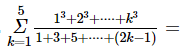Test: BITSAT Mock Test- 2 - Question 99

If A and B are square matrices and A⁻1 and B⁻1 of same order exists, then (AB)⁻1 is equal to

Detailed Solution for Test: BITSAT Mock Test- 2 - Question 99

B⁻1A⁻1.

Explanation:

Given that A and B are square matrices and their inverses A⁻1 and B⁻1 exist, we want to find the inverse of the product AB, denoted as (AB)⁻1.

To show that (AB)⁻1 = B⁻1A⁻1, we will demonstrate that (AB)(B⁻1A⁻1) = I and (B⁻1A⁻1)(AB) = I, where I is the identity matrix.

First, let's find the product (AB)(B⁻1A⁻1):

(AB)(B⁻1A⁻1) = A(BB⁻1)A⁻1 (associative property)

Since B and B⁻1 are inverses, their product BB⁻1 is the identity matrix:

A(IA⁻1) = A(A⁻1)

And because A and A⁻1 are inverses, their product AA⁻1 is the identity matrix:

Test: BITSAT Mock Test- 2 - Question 100

if A is a 3 x 3 matrix and B is its adjoint matrix. If ∣B∣ = 64, then ∣A∣ =

Test: BITSAT Mock Test- 2 - Question 101

If α , β are the roots of the quadratic equation x2 − (a − 2)  x − (a + 1)  = 0 , where a is a variable, then the least value of α2 + β2 is

Test: BITSAT Mock Test- 2 - Question 102

The point represented by the complex number 2 - i is rotated about origin through an angle of π/2 in clockwise direction. The new position of the point is

Test: BITSAT Mock Test- 2 - Question 103

A quadrilateral is formed by pairs of lines xy+x+y+1=0, xy+3x+3y+9=0, then quadrilateral is

Test: BITSAT Mock Test- 2 - Question 104

Find the equation of the normal to curve x2=4y which passes through the point (1, 2).

Detailed Solution for Test: BITSAT Mock Test- 2 - Question 104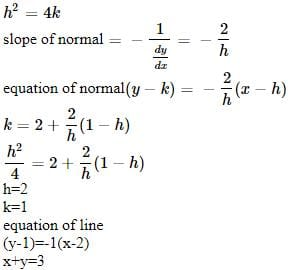Test: BITSAT Mock Test- 2 - Question 105

On the parabola y2 = 8 x if one extremity of a focal chord is 1 ∕ 2, − 2 then its other extremity is

Test: BITSAT Mock Test- 2 - Question 106

If nPr=840, nCr=35, then n=

Detailed Solution for Test: BITSAT Mock Test- 2 - Question 106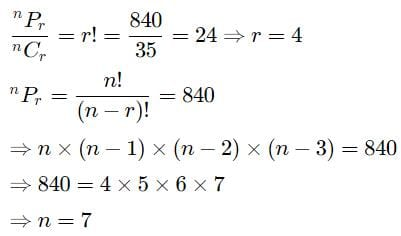Test: BITSAT Mock Test- 2 - Question 107

We have (n+1) white balls and (n+1) black balls. In each set the balls are numbered from 1 to (n+1). If these balls are to be arranged in a row so that two consecutive balls are of different colours, then the number of these arrangements is

Detailed Solution for Test: BITSAT Mock Test- 2 - Question 107

Between (n + 1) white balls there are (n + 2) gaps in which (n + 1) black ball are to arranged.
No. of reqd arrangements = (n + 1)! (n + 1)! = [(n + 1)!]2
Now between (n + 1) black balls (n + 1) white balls are to be filled no. of ways
= (n + 1)! (n + 1)!
∴ Reqd ways = 2[(n + 1)!]2

Test: BITSAT Mock Test- 2 - Question 108

An urn contains 9 balls two of which are red, three blue and four black. Three balls are drawn at random. The probability that they are of same colour is

Test: BITSAT Mock Test- 2 - Question 109

A coin is tossed successively three times. The probability of getting at least 2 heads is:

Test: BITSAT Mock Test- 2 - Question 110

In ΔABC , if a,b,c, are in A. P., then tan A/2 tan C/2 =

Test: BITSAT Mock Test- 2 - Question 111

In a Δ A B C , A = 90o . Then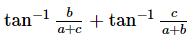is equal to

Test: BITSAT Mock Test- 2 - Question 112

8 sec2 θ - 6 sec θ + 1 = 0 has

Test: BITSAT Mock Test- 2 - Question 113

The sum of infinite terms of the G.P. √2 + 1/√2 - 1, 1/2 - √2, 1/2,... is

Test: BITSAT Mock Test- 2 - Question 114

If the sum of the first n terms of a series be 5n2 + 2n, then its second term is

Test: BITSAT Mock Test- 2 - Question 115

Let N denote the set of all natural numbers and R be the relation on N x N defined by (a,b)R(c,d) if ad(b+c)=bc(a+d), then R is

Test: BITSAT Mock Test- 2 - Question 116

If A and B are matrices of order 2 such that A * B = (AB - BA)/2 then which of the following is false?

Test: BITSAT Mock Test- 2 - Question 117

The equation of line passing through lines 5x-6y-1=0 and 3x+2y+5=0 and perpendicular to 3x-5y+11=0 is

Test: BITSAT Mock Test- 2 - Question 118

The angle between the planes 2x - y + z = 6 and x + y + 2z = 7 is

Test: BITSAT Mock Test- 2 - Question 119

The length of the subtangent to the curve x2+xy+y2=7 at (1,-3) is

Test: BITSAT Mock Test- 2 - Question 120

The equation of the sphere passing thro' the point (1,3-2) and the circle y2+z2=25 and x=0 is

Test: BITSAT Mock Test- 2 - Question 121

If tanθ+secθ=√3, 0<θ<π, then θ=

Test: BITSAT Mock Test- 2 - Question 122

The general value of θ which satisfies tanθ=-1 and cosθ=(1/√2) the equations is

Test: BITSAT Mock Test- 2 - Question 123

[(2 sinθ tanθ(1-tanθ)+2 sinθ sec2θ)/(1+tanθ)2]=

Test: BITSAT Mock Test- 2 - Question 124

The perimeter of a triangle formed by 3i+4j+5k, 4i-3j-5k and 7i+j is

Detailed Solution for Test: BITSAT Mock Test- 2 - Question 124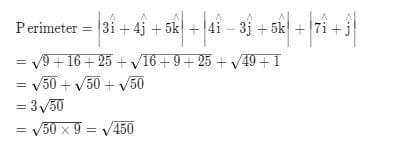Test: BITSAT Mock Test- 2 - Question 125

If |a|=3, |b|=4, |c|=5 are such that each is perpendicular to the sum of other two, then |a+b+c|=

Test: BITSAT Mock Test- 2 - Question 126

Find the Antonym of the word occurring in the sentence in capital letter as per the context.
The two friends were DISTINCT in everything; dress manners, hair-style and food-habits.

Detailed Solution for Test: BITSAT Mock Test- 2 - Question 126

DISTINCT here means 'different'

Test: BITSAT Mock Test- 2 - Question 127

Find the Antonym of the word occurring in the sentence in capital letter as per the context.
For the first time I saw him speaking RUDELY to her

Detailed Solution for Test: BITSAT Mock Test- 2 - Question 127

'Rudely' means showing lack of respect.
So its antonym will be Politely

Test: BITSAT Mock Test- 2 - Question 128

Fill in the blank with appropriate word.
In hot weather I like lying in the........of a tree.

Test: BITSAT Mock Test- 2 - Question 129

Fill in the blank with appropriate word.
This was the first time Roshan had been found guilty. He has no.......convictions

Test: BITSAT Mock Test- 2 - Question 130

Choose the one which can be substituted for the given words/sentences
The policy of extending a country's empire and influence

Test: BITSAT Mock Test- 2 - Question 131

Choose the one which can be substituted for the given words/sentences
A person who insists on something.

Test: BITSAT Mock Test- 2 - Question 132

Spot the error.

Test: BITSAT Mock Test- 2 - Question 133

Spot the error.

Test: BITSAT Mock Test- 2 - Question 134

Spot the error.

Detailed Solution for Test: BITSAT Mock Test- 2 - Question 134

Yet, firms and other concerns are spending large sums of money all the time

Test: BITSAT Mock Test- 2 - Question 135

Find the Synonym of CAVIL.

Test: BITSAT Mock Test- 2 - Question 136

Find the Synonym of the word occurring in the sentence in capital letter as per the context.
He is very intelligent, but ILL-FAVOURED by nature

Detailed Solution for Test: BITSAT Mock Test- 2 - Question 136

Meaning of ILL-FAVOUR - bad tempered, intended meaning here is - She is intelligent but not good at her conduct

Test: BITSAT Mock Test- 2 - Question 137

Choose the word which is least like the other words in the group .

Test: BITSAT Mock Test- 2 - Question 138

Complete the analogous pair.
Earth : Sun : : Moon : ?

Test: BITSAT Mock Test- 2 - Question 139

Group the following figures into three classes on the basis of identical properties.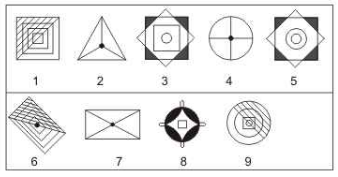Detailed Solution for Test: BITSAT Mock Test- 2 - Question 139

1,6,9 are figures which are half shaded by slanting lines
2,4,7 are all divided into equal parts (either three or four parts) by straight lines and also have a black circle at the centre.
3,5,8 have similar designs and have their four corners shaded black.

Test: BITSAT Mock Test- 2 - Question 140

Find the missing character.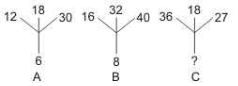Detailed Solution for Test: BITSAT Mock Test- 2 - Question 140

The above three numbers are multiples of the number at the bottom.
Clearly, 36, 18 and 27 are all multiples of 9.
So, the missing number is 9.

Test: BITSAT Mock Test- 2 - Question 141

In each question, three sequences of letters/numerals are given which correspond to each other. Find out the letters/numerals that come in vacant places.
A _ B A C _ D _ B C D C
_ 3 _ 2 _ 1 _ 4 ? ? ? ?
d c _ _ b a c b _ _ _ _

Test: BITSAT Mock Test- 2 - Question 142

In this letter series, some of the letters are missing. Choose the correct letter given below -
ab _ aa _ caab _ c _ abb _ c

Test: BITSAT Mock Test- 2 - Question 143

The practice of appointing educated persons to join under government began more than a hundred and thirty years ago as a method of encouraging people to go in for the new system of education that had been established by the colonial administration. It worked well till about 1921 because expansion of jobs under the government could still keep pace with the output of the educational system. But since then, the undesirable consequences of this approach have come to the fore. To begin with, it over emphasizes the bread and butter objective of education and makes it a ladder which enables the ambitious to climb into privilege, even as it devalues the academic objective-the development of individuals dedicated to the pursuit of scholarship. Moreover, hunt for a place in the privileged sector or a well-paid and secure job in the organized sector creates immense pressure for the expansion of secondary and higher education because they grow more in terms of the large numbers of aspirants for jobs rather than in relation to the small number of jobs available. This necessarily leads to an over-production of college graduates and to large-scale unemployment of the educated.
Which one of the following statements is correct?

Educated persons were given jobs under the government, more than a century ago, so that the young people

Test: BITSAT Mock Test- 2 - Question 144

The practice of appointing educated persons to join under government began more than a hundred and thirty years ago as a method of encouraging people to go in for the new system of education that had been established by the colonial administration. It worked well till about 1921 because expansion of jobs under the government could still keep pace with the output of the educational system. But since then, the undesirable consequences of this approach have come to the fore. To begin with, it over emphasizes the bread and butter objective of education and makes it a ladder which enables the ambitious to climb into privilege, even as it devalues the academic objective-the development of individuals dedicated to the pursuit of scholarship. Moreover, hunt for a place in the privileged sector or a well-paid and secure job in the organized sector creates immense pressure for the expansion of secondary and higher education because they grow more in terms of the large numbers of aspirants for jobs rather than in relation to the small number of jobs available. This necessarily leads to an over-production of college graduates and to large-scale unemployment of the educated.
Which one of the following statements is correct?

129. Which one of the following statements is correct?
The policies of the colonial administration were effective till about 1921 because

Test: BITSAT Mock Test- 2 - Question 145

The practice of appointing educated persons to join under government began more than a hundred and thirty years ago as a method of encouraging people to go in for the new system of education that had been established by the colonial administration. It worked well till about 1921 because expansion of jobs under the government could still keep pace with the output of the educational system. But since then, the undesirable consequences of this approach have come to the fore. To begin with, it over emphasizes the bread and butter objective of education and makes it a ladder which enables the ambitious to climb into privilege, even as it devalues the academic objective-the development of individuals dedicated to the pursuit of scholarship. Moreover, hunt for a place in the privileged sector or a well-paid and secure job in the organized sector creates immense pressure for the expansion of secondary and higher education because they grow more in terms of the large numbers of aspirants for jobs rather than in relation to the small number of jobs available. This necessarily leads to an over-production of college graduates and to large-scale unemployment of the educated.
Which one of the following statements is correct?

Which one of the following statements is correct?
The system failed later on because

Test: BITSAT Mock Test- 2 - Question 146

The practice of appointing educated persons to join under government began more than a hundred and thirty years ago as a method of encouraging people to go in for the new system of education that had been established by the colonial administration. It worked well till about 1921 because expansion of jobs under the government could still keep pace with the output of the educational system. But since then, the undesirable consequences of this approach have come to the fore. To begin with, it over emphasizes the bread and butter objective of education and makes it a ladder which enables the ambitious to climb into privilege, even as it devalues the academic objective-the development of individuals dedicated to the pursuit of scholarship. Moreover, hunt for a place in the privileged sector or a well-paid and secure job in the organized sector creates immense pressure for the expansion of secondary and higher education because they grow more in terms of the large numbers of aspirants for jobs rather than in relation to the small number of jobs available. This necessarily leads to an over-production of college graduates and to large-scale unemployment of the educated.
Which one of the following statements is correct?

The expansion of secondary and higher education leads to which one of the following?

Test: BITSAT Mock Test- 2 - Question 147

Select the correct mirror image of the figure (X) from amongst the given alternatives.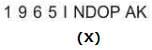Test: BITSAT Mock Test- 2 - Question 148

Select the correct mirror image of the figure (X) from amongst the given alternatives.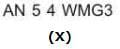Test: BITSAT Mock Test- 2 - Question 149

Each of the following questions consists of five Problem figures marked 1,2,3,4 and 5 followed by five Answer figures marked A,B,C,D and E . Select a figure from the Answer figures which will continue the same series as given in the Problem figures.